# How to get a list of unique and distinct values in Excel

This is the final part of the Excel Unique Values series that shows how to get a list of distinct / unique values in column using a formula, and how to tweak that formula for different datasets. You will also learn how to quickly get a distinct list using Excel's Advanced Filter, and how to extract unique rows with Duplicate Remover.

In a couple of recent articles, we discussed different methods to count and find unique values in Excel. If you had a chance to read those tutorials, you already know how to get a unique or distinct list by identifying, filtering, and copying. But that's a bit long, and by far not the only, way to extract unique values in Excel. You can do it much faster by using a special formula, and in a moment I will show you this and a couple of other techniques.

Tip. To quickly get unique values in the latest version of Excel 365 that supports dynamic arrays, use the UNIQUE function as explained in the above linked tutorial.

## How to get unique values in Excel

To avoid any confusion, first off, let's agree on what we call unique values in Excel. Unique values are the values that exist in a list only once. For example: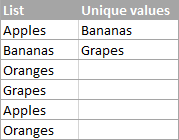To extract a list of unique values in Excel, use one of the following formulas.

Array unique values formula (completed by pressing Ctrl + Shift + Enter):

`=IFERROR(INDEX(\$A\$2:\$A\$10, MATCH(0, COUNTIF(\$B\$1:B1,\$A\$2:\$A\$10) + (COUNTIF(\$A\$2:\$A\$10, \$A\$2:\$A\$10)<>1), 0)), "")`

Regular unique values formula (completed by pressing Enter):

`=IFERROR(INDEX(\$A\$2:\$A\$10, MATCH(0,INDEX(COUNTIF(\$B\$1:B1, \$A\$2:\$A\$10)+(COUNTIF(\$A\$2:\$A\$10, \$A\$2:\$A\$10)<>1),0,0), 0)), "")`

In the above formulas, the following references are used:

• A2:A10 - the source list.
• B1 - the top cell of the unique list minus 1. In this example, we start the unique list in B2, and therefore we supply B1 to the formula (B2-1=B1). If your unique list begins, say, in cell C3, then change \$B\$1:B1 to \$C\$2:C2.
Note. Because the formula references the cell above the first cell of the unique list, which is usually the column header (B1 in this example), make sure your header has a unique name that does not appear anywhere else in the column.

In this example, we are extracting unique names from column A (more precisely from range A2:A20), and the following screenshot demonstrates the array formula in action: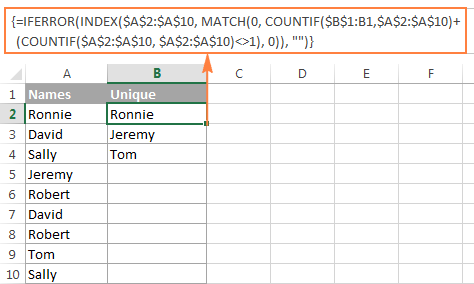The detailed explanation of the formula's logic is provided in a separate section, and here's how to use the formula to extract unique values in your Excel worksheets:

• Tweak one of the formulas according to your dataset.
• Enter the formula in the first cell of the unique list (B2 in this example).
• If you are using the array formula, press Ctrl + Shift + Enter. If you've opted for the regular formula, press the Enter key as usual.
• Copy the formula down as far as needed by dragging the fill handle. Since both unique values formulas are we encapsulated in the IFERROR function, you can copy the formula up to the end of your table, and it won't clutter your data with any errors no matter how few unique values have been extracted.

## How to get distinct values in Excel (unique + 1st duplicate occurrences)

As you may have already guessed from the heading of this section, distinct values in Excel are all different values in a list, i.e. unique values and first instances of duplicate values. For example: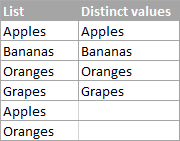To get a distinct list in Excel, use the following formulas.

Array distinct formula (requires pressing Ctrl + Shift + Enter):

`=IFERROR(INDEX(\$A\$2:\$A\$10, MATCH(0, COUNTIF(\$B\$1:B1, \$A\$2:\$A\$10), 0)), "")`

Regular distinct formula:

`=IFERROR(INDEX(\$A\$2:\$A\$10, MATCH(0, INDEX(COUNTIF(\$B\$1:B1, \$A\$2:\$A\$10), 0, 0), 0)), "")`

Where:

• A2:A10 is the source list.
• B1 is the cell above the first cell of the distinct list. In this example, the distinct list begins in cell B2 (it's the first cell where you enter the formula), so you reference B1.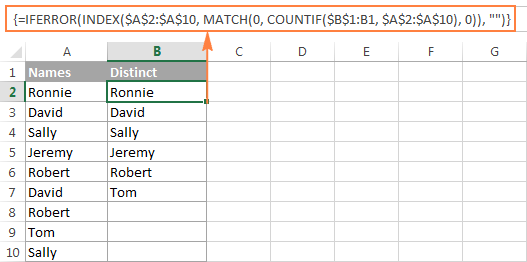### Extract distinct values in a column ignoring blank cells

If your source list contains any blank cells, the distinct formula we've just discussed would return a zero for each empty row, which might be a problem. To fix this, improve the formula a bit further:

Array formula to extract distinct values excluding blanks:

`=IFERROR(INDEX(\$A\$2:\$A\$10, MATCH(0, COUNTIF(\$B\$1:B1, \$A\$2:\$A\$10&"") + IF(\$A\$2:\$A\$10="",1,0), 0)), "")`

### Get a list of distinct text values ignoring numbers and blanks

In a similar manner, you can get a list of distinct values excluding empty cells and cells with numbers:

`=IFERROR(INDEX(\$A\$2:\$A\$10, MATCH(0, COUNTIF(\$B\$1:B1, \$A\$2:\$A\$10&"") + IF(ISTEXT(\$A\$2:\$A\$10)=FALSE,1,0), 0)), "")`

As a quick reminder, in the above formulas, A2:A10 is the source list, and B1 is cell right above the first cell of the distinct list.

The following screenshot shows the result of both formulas: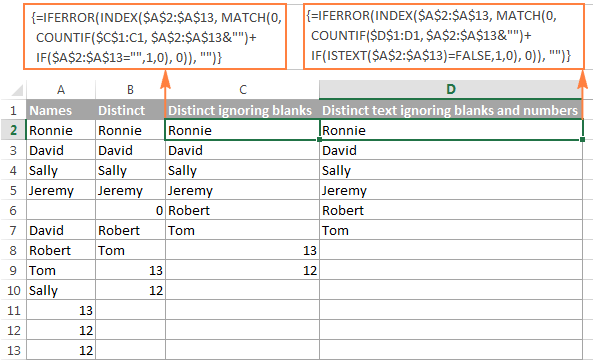### How to extract case-sensitive distinct values in Excel

When working with case-sensitive data such as passwords, user names or file names, you may need to get a list of case-sensitive distinct values. For this, use the following array formula, where A2:A10 is the source list, and B1 is the cell above the first cell of the distinct list:

Array formula to get case-sensitive distinct values (requires pressing Ctrl + Shift + Enter)

`=IFERROR(INDEX(\$A\$2:\$A\$10, MATCH(0, FREQUENCY(IF(EXACT(\$A\$2:\$A\$10,TRANSPOSE(\$B\$1:B1)), MATCH(ROW(\$A\$2:\$A\$10), ROW(\$A\$2:\$A\$10)), ""), MATCH(ROW(\$A\$2:\$A\$10), ROW(\$A\$2:\$A\$10))), 0)), "")`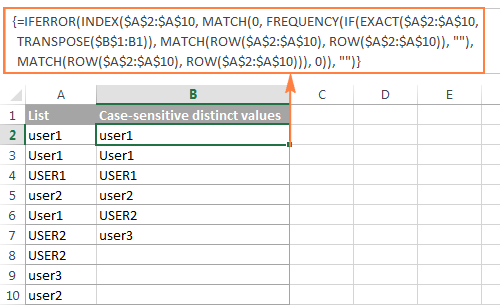## How the unique / distinct formula works

This section is written especially for those curious and thoughtful Excel users who not only want to know the formula but fully understand its nuts and bolts.

It goes without saying that the formulas to extract unique and distinct values in Excel are neither trivial nor straightforward. But having a closer look, you may notice that all the formulas are based on the same approach - using INDEX/MATCH in combination with COUNTIF, or COUNTIF + IF functions.

For our in-depth analysis, let's use the array formula that extracts a list of distinct values because all other formulas discussed in this tutorial are improvements or variations of this basic one:

`=IFERROR(INDEX(\$A\$2:\$A\$10, MATCH(0, COUNTIF(\$B\$1:B1, \$A\$2:\$A\$10), 0)), "")`For starters, let's cast away the obvious IFERROR function, which is used with a single purpose to eliminate #N/A errors when the number of cells where you've copied the formula exceeds the number of distinct values in the source list.

And now, let's break down the core part of our distinct formula:

1. COUNTIF(range, criteria) returns the number of cells within a range that meet a specified condition.

In this example, COUNTIF(\$B\$1:B1, \$A\$2:\$A\$10) returns an array of 1's and 0's based on whether any of the values of the source list (\$A\$2:\$A\$10) appears somewhere in the distinct list (\$B\$1:B1). If the value is found, the formula returns 1, otherwise - 0.

In particular, in cell B2, COUNTIF(\$B\$1:B1, \$A\$2:\$A\$10) becomes:

`COUNTIF("Distinct", {"Ronnie"; "David"; "Sally"; "Jeremy"; "Robert"; "David"; "Robert"; "Tom"; "Sally"})`

and returns:

`{0;0;0;0;0;0;0;0;0}`

because none of the items of the source list (criteria) appears in the range where the function looks for a match. In this case, range (\$B\$1:B1) consists of a single item - "Distinct".

2. `MATCH(lookup_value, lookup_array, [match_type])` returns the relative position of the lookup value in the array.
In this example, the lookup_value is 0, and consequently:

`MATCH(0,COUNTIF(\$B\$1:B1, \$A\$2:\$A\$10), 0)`

turns into:

`MATCH(0, {0;0;0;0;0;0;0;0;0},0)`

and returns 1

because our MATCH function gets the first value that is exactly equal to the lookup value (as you remember, the lookup value is 0).

3. INDEX(array, row_num, [column_num]) returns a value in an array based on the specified row and (optionally) column numbers.

In this example, INDEX(\$A\$2:\$A\$10, 1)

becomes:

`INDEX({"Ronnie"; "David"; "Sally"; "Jeremy"; "Robert"; "David"; "Robert"; "Tom"; "Sally"}, 1)`

and returns "Ronnie".

When the formula is copied down the column, the distinct list (\$B\$1:B1) expands because the second cell reference (B1) is a relative reference that changes according to the relative position of the cell where the formula moves.

So, when copied to cell B3, COUNTIF(\$B\$1:B1, \$A\$2:\$A\$10) changes to COUNTIF(\$B\$1:B2, \$A\$2:\$A\$10), and becomes:

`COUNTIF({"Distinct";"Ronnie"}, {"Ronnie"; "David"; "Sally"; "Jeremy"; "Robert"; "David"; "Robert"; "Tom"; "Sally"}), 0)), "")`

and returns:

`{1;0;0;0;0;0;0;0;0}`

because one "Ronnie" is found in range \$B\$1:B2.

And then, MATCH(0,{1;0;0;0;0;0;0;0;0},0) returns 2, because 2 is the relative position of the first 0 in the array.

And finally, `INDEX(\$A\$2:\$A\$10, 2)` returns the value from the 2nd row, which is "David".

Tip. For better understanding of the formula's logic, you can select different parts of the formula in the formula bar and press F9 to see what a selected part evaluates to: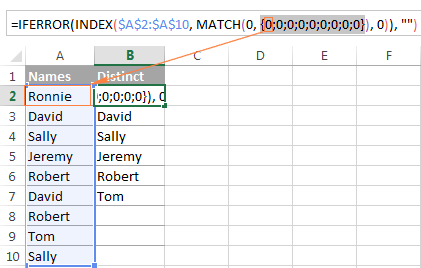If you still have difficulties figuring out the formula, you can check out the following tutorial for the detailed explanation of how the INDEX/MATCH liaison works: INDEX & MATCH as a better alternative to Excel VLOOKUP.

As already mentioned, the other formulas discussed in this tutorial are based on the same logic, with just a few modifications:

Unique values formula - contains one more COUNTIF function that excludes from the unique list all items that appear in the source list more than once: `COUNTIF(\$A\$2:\$A\$10, \$A\$2:\$A\$10)<>1`.

Distinct values formula ignoring blanks - here you add an IF function that prevents blank cells from being added to the distinct list: `IF(\$A\$2:\$A\$13="",1,0)`.

Distinct text values formula ignoring numbers - you use the ISTEXT function to check whether a value is text, and the IF function to dismiss all other value types, including blank cells: `IF(ISTEXT(\$A\$2:\$A\$13)=FALSE,1,0)`.

## Extract distinct values from a column with Excel's Advanced Filter

If you don't want to waste time on figuring out the arcane twists of the distinct value formulas, you can quickly get a list of distinct values by using the Advanced Filter. The detailed steps follow below.

1. Select the column of data from which you want to extract distinct values.
2. Switch to the Data tab > Sort & Filter group, and click the Advanced button: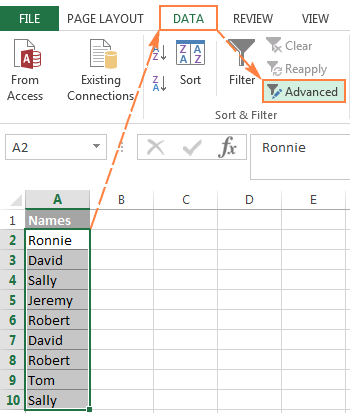3. In the Advanced Filter dialog box, select the following options:
• Check Copy to another location radio button.
• In the List range box, verify that the source range is displayed correctly.
• In the Copy to box, enter the topmost cell of the destination range. Please keep in mind that you can copy the filtered data only to the active sheet.
• Select the Unique records only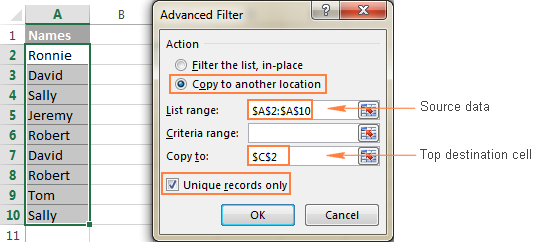4. Finally, click the OK button and check the result: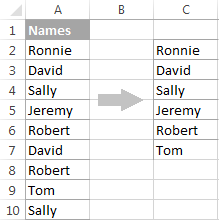Please pay attention that although the Advanced Filter's option is named "Unique records only", it extracts distinct values, i.e. unique values and 1st occurrences of duplicate values.

## Extract unique and distinct rows with Duplicate Remover

In the final part of this tutorial, let me show you our own solution to find and extract distinct and unique values in Excel sheets. This solution combines the versatility of Excel formulas and simplicity of the advanced filter. In addition, it provides a couple of unique features such as:

• Find and extract unique / distinct rows based on values in one or more columns.
• Find, highlight, and copy unique values to any other location, in the same or different workbook.

And now, let's see the Duplicate Remover tool in action.

Supposing you have a summary table created by consolidating data from several other tables. Obviously, that summary table contains a lot of duplicate rows and your task is to extract unique rows that appear in the table only once, or distinct rows including unique and 1st duplicate occurrences. Either way, with the Duplicate Remover add-in the job is done in 5 quick steps.

1. Select any cell within your source table and click the Duplicate Remover button on the Ablebits Data tab, in the Dedupe group.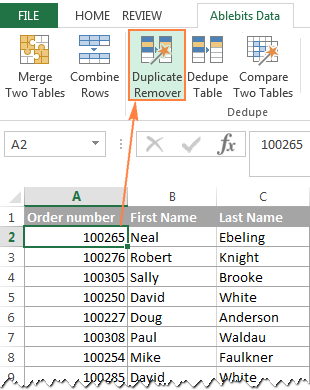The Duplicate Remover wizard will run and select the entire table. So, just click Next to proceed to the next step.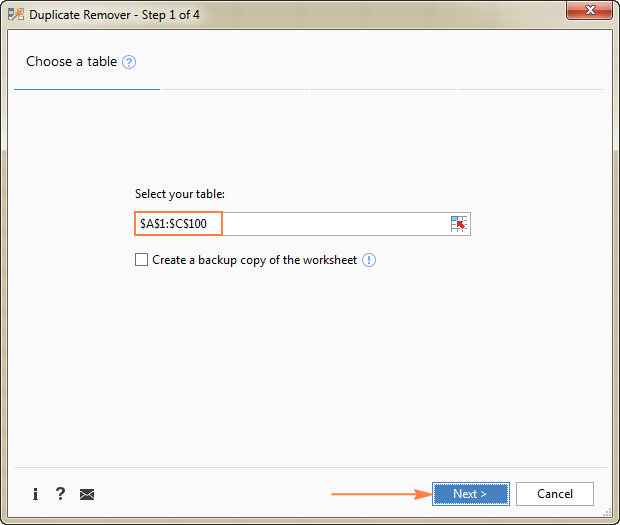2. Select the value type you want to find, and click Next:
• Unique
• Unique +1st occurrences (distinct)

In this example, we aim to extract unique rows that appear in the source table only once, so we select the Unique option: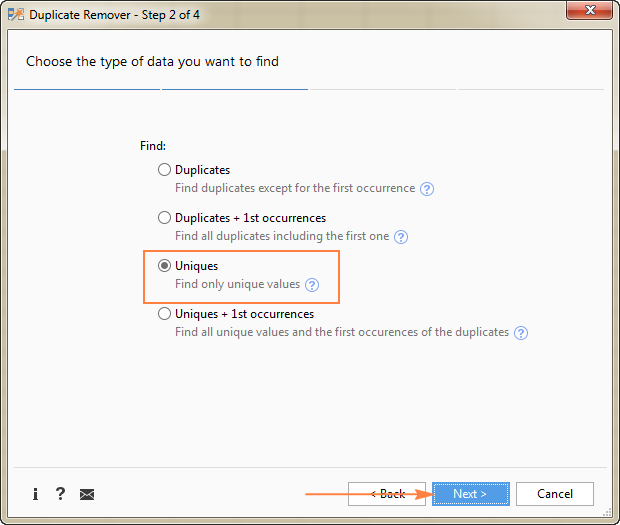Tip. As you can see in the above screenshot, there are also 2 options for duplicate values, just keep it in mind if you need to dedupe some other worksheet.
3. Select one or more columns to be checked for unique values.

In this example, we want to find unique rows based on values in all 3 columns (Order number, First name and Last name), therefore we select all.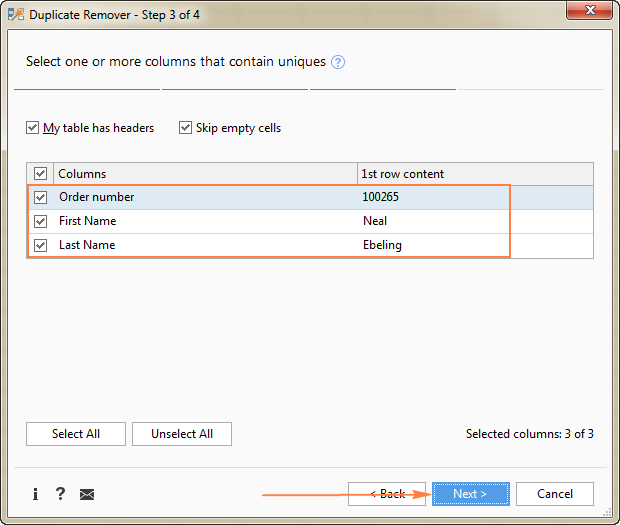4. Choose the action to perform on the found unique values. The following options are available to you:
• Highlight unique values
• Select unique values
• Identify in a status column
• Copy to another location

Because we are extracting unique rows, select Copy to another location, and then specify where exactly you want to copy them - active sheet (select the Custom location option, and specify the top cell of the destination range), new worksheet or new workbook.

In this example, let's opt for the new sheet: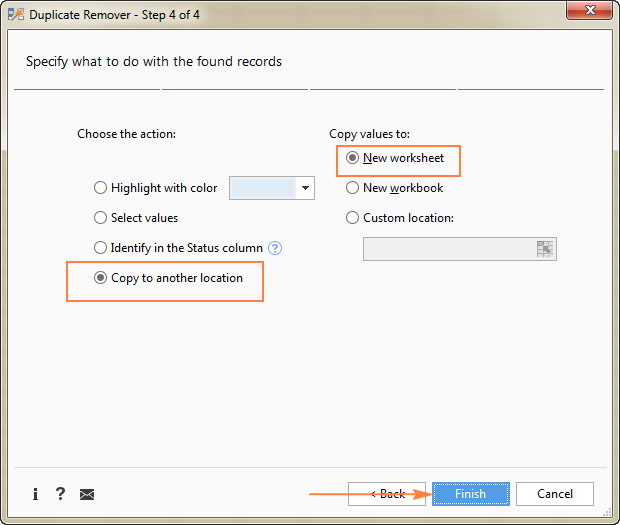5. Click the Finish button, and you are done!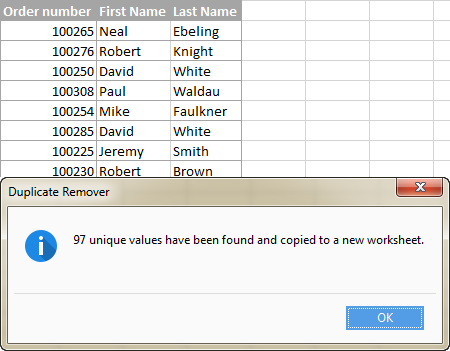Liked this quick and simple way to get a list of unique values or rows in Excel? If so, I encourage you to download an evaluation version below and give it a try. Duplicate Remover as well as all other time-saving tools that we have are included with Ultimate Suite for Excel.

Find Unique Values in Excel - sample workbook (.xlsx file)
Ultimate Suite - evaluation version (.zip file)

## You may also be interested in

### 142 comments to "How to get a list of unique and distinct values in Excel"

1.Dawid Pintal says:

Small update to my question

I am trying just something very simple as table:
Just 2 columns: Names | UNIQUE
In the column Names we have "texts" as below
00340312891320582291
00340312891920067756
00340312891920069262
00340312891320580598
00340312891320578410
00340312891320578189
00340312891320578854
00340312891320577890
00340312891320578231
00340312891320577661
In the column UNIQUE - should appear only unique values, in this example everything is unique but to compare it it must be as text not numbers like all the time.
Ignore please concatenate in my message before, its just trying to find a way. I clearly use your description and it not works for this example - only when I add prefix as letter or something :(
Help please - from one column to another just unique, especially if it can works with Table - area defined as table

Thanks,
Dawid

•Alexander Trifuntov (Ablebits Team) says:

Hello!
If I understand correctly, you want to keep leading zeros in numbers. An easy way to preserve leading zeros in Excel is to precede the number with an apostrophe ('). For example, instead of entering 0034, enter '0034.
Without this, Excel will ignore leading zeros and will convert your text to a number.
The following tutorial should help: How to keep leading zeros in Excel.

2.Dawid Pintal says:

Hello,
thank you for good advice in Distinct extraction problem, but cannot find a solution with probably bug in Excel. It is about specific text values which actually looks like numbers - but it isn't.

For example I have column names as below (all of it is text, not numbers, without ' ):
Names
00340312891320582291
00340312891920067756
00340312891920069262
00340312891320580598
00340312891320578410
00340312891320578189
00340312891320578854
00340312891320577890
00340312891320578231
00340312891320577661

when I use formula (normal or array) as in your example above:
=IFERROR(INDEX(CONCATENATE(\$A\$2:\$A\$10),MATCH(0,COUNTIF(\$B\$1:B1,\$A\$2:\$A\$10),0)),"")
it skip many values - taking it as numbers I think. Result is:

Distinct
00340312891320582291
00340312891920067756
00340312891920069262
00340312891320580598
00340312891320578410
00340312891320577890

but all of my input data is unique so how can it be? I just want to take it all as text or numbers - probably excel change it in this formula to the scientific format and some numbers after transformation is the same. This is what I think, excel used here to compare and give bad result:
Names
340312891320582000
340312891920067000
340312891920069000
340312891320580000
340312891320578000
340312891320578000
340312891320578000
340312891320577000
340312891320578000
340312891320577000

and in this compare result is like above - just 6 "Names" appear rest of them is the same, all because of scientific format used here to compare not as a texts. Problem disappear when my input is with any letter as prefix (like: "a00340312891320582291"). So it is no more number (probably too long for amateur windows software) and its okay, I have 10 results as a source, nothing disappear.

I am really confused how to skip it, just compare text to text as it is not a numbers

Why up to the office version 2021 this problem is not resolved, there is really no function to extract it easy - not pivot table, I know it can be and okay, but I don't want make to many temporary references. Just normal function - take from one range, unique values to another range. The best is normal Table defined by Control+T and using columns names not range like [Names] or [Distinct].

Is it any way possible?

3.David Robins says:

Regarding my SORT question, I found that even using the manual sorting functions in Excel also do nothing to the unique column, which is presumably why the SORT function doesn’t work. I suspect sorting these cells that have the formula still results in the finding of the unique data list in its original order. My compromise is to write the SORT in the adjacent column which works.

Another solution is to sort the data before using the unique extraction.

4.David Robins says:

Great teaching. Thanks.

I’d like to get the unique alphabetically sorted.

Using Excel 365’s SORT function in various places in the first formula you show, I can’t get SORT to do anything.

Is that because the SORT function has to happen after the list is extracted, and there is no way to set the order of the functions?

Thanks.

•Robins David says:

Regarding trying to use the SORT function combined with the unique lost process:

I tried just a manual Sort on the unique column, but nothing seemed to happen. It’s possible it did soft the formula cells but it returned with the same list, first found-last found. Probably because it is going back to the data and finding it again in the same order.

FILTER function wouldn’t help with this unique formula as there is nothing to filter until it is all done. (I assume.)

So, I could sort the source data. But will just continue with a helped column where the SORT function sorts the unique list. Easier since it is now programmed in, no macros needed.

5.Erik says:

I am trying to apply this to a book inventory list for my library.
I'd like to setup a unique list in one column with the number of copies for every book we have in the inventory. Currently our system only shows us the overall total of books, but not how many of each copy we have, so we have several copies within our database but would need to manually count them in excel which simply isn't efficient. I was toying a bit with the built in advanced function earlier and it works to some degree, however, I did notice that when expanding this to have multiple criteria's to watch out for, it would count some of the items in the list twice and the overall total would be off. The sheet I'm working with has 1187 total entries, but the advanced formula using unique title and author has a result of 1211 total entries.

•Alexander Trifuntov (Ablebits Team) says:

Hello!
I don't know what formula you used. I recommend checking out this guide: Count unique values with criteria.